# Ch 3 final ppt

27. Sep 2010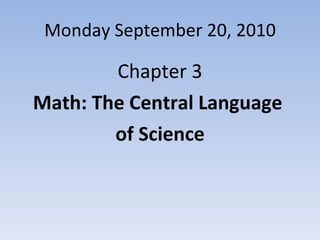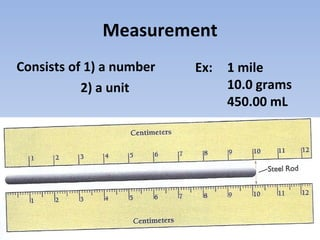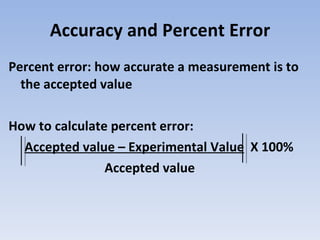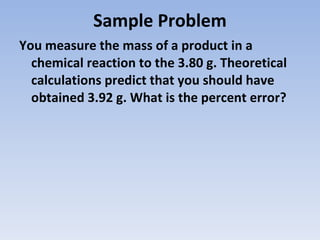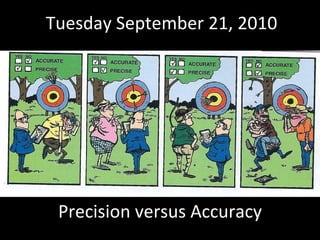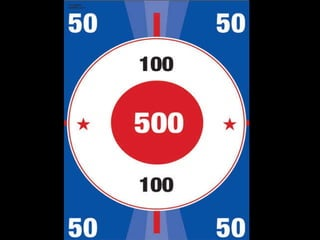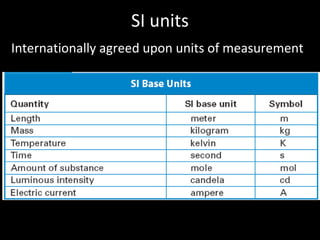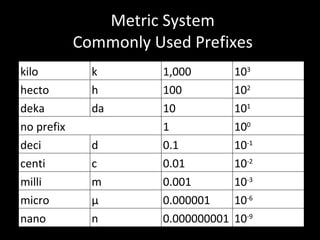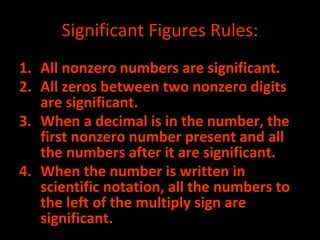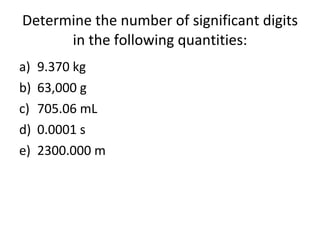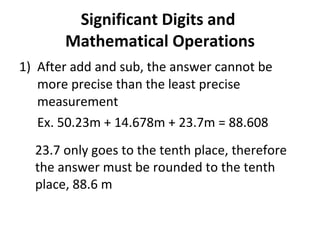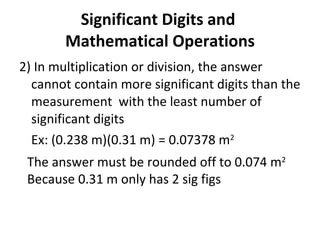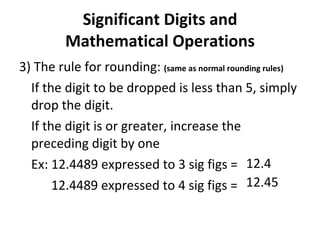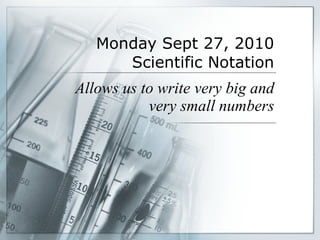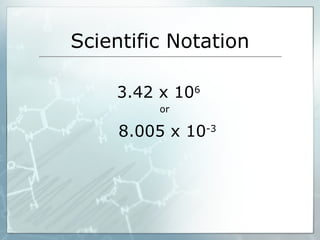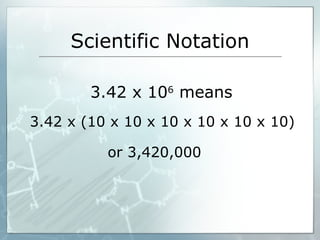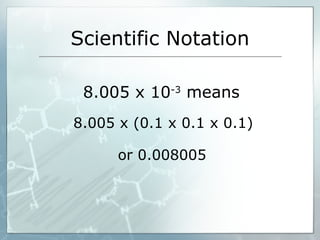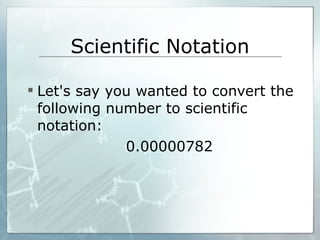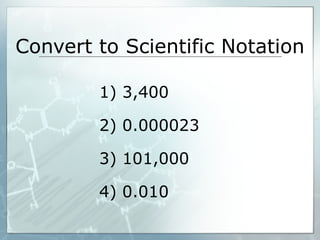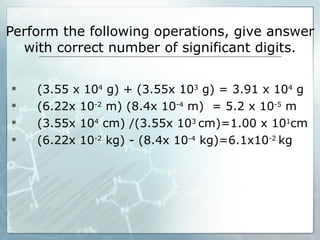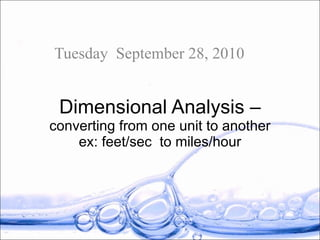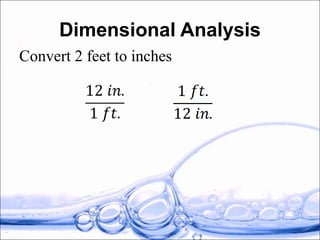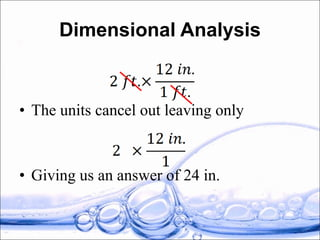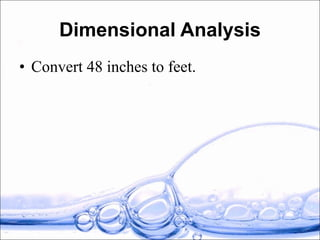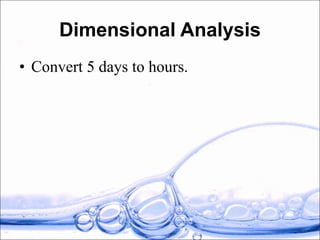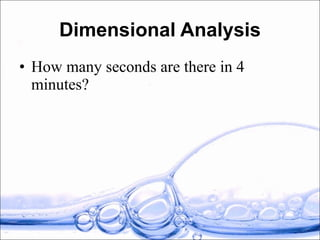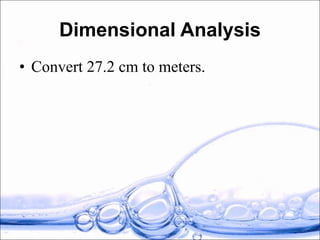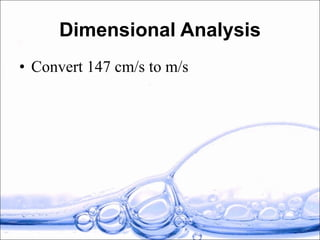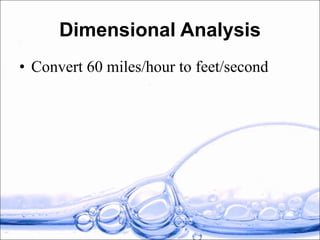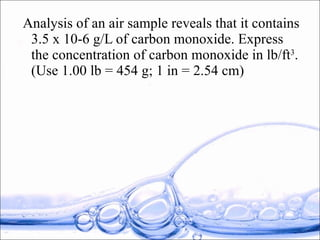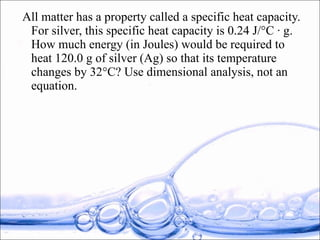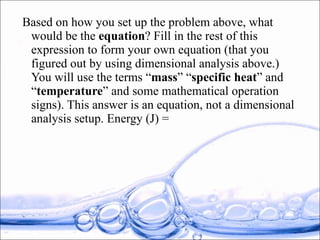1 von 33

### Ch 3 final ppt

• 1.
• 2.
• 3.
• 4.
• 5.
• 6.
• 7.
• 8. Metric System Commonly Used Prefixes kilo k 1,000 10 3 hecto h 100 10 2 deka da 10 10 1 no prefix 1 10 0 deci d 0.1 10 -1 centi c 0.01 10 -2 milli m 0.001 10 -3 micro µ 0.000001 10 -6 nano n 0.000000001 10 -9
• 9. Significant Figures Made Easy By Miss Virginia Williams
• 10.
• 11.
• 12.
• 13.
• 14.
• 15. Monday Sept 27, 2010 Scientific Notation Allows us to write very big and very small numbers
• 16.
• 17.
• 18.
• 19.
• 20.
• 21.
• 22. Dimensional Analysis – converting from one unit to another ex: feet/sec to miles/hour Tuesday September 28, 2010
• 23.
• 24.
• 25.
• 26.
• 27.
• 28.
• 29.
• 30.
• 31.
• 32.
• 33.

### Hinweis der Redaktion

1. Measurements are made up of both accuracy and precision Accuracy, how close the measured value is to the accepted value Precision, how finely divided the measurement scale is on the device
2. How close a measurement is to the accepted value When ever a measurement is taken, there is always a chance for human error
3. How finely divided the measurement scale is on an instrument Repeated measurements should yield nearly identical values A set of measurements can be accurate without being precise, precise without being accurate, neither accurate nor precise, or both accurate and precise
4. All scientists use these to measure
5. All units are multiples of ten Consist of base unit and prefix
6. Give students handout with these rules
7. Every number written in scientific notation has two basic parts.  The first part is always a decimal number between 1.00 and 9.99… The second part is 10 times some exponent Notice that in each of these, the first part is a decimal number between 1.00 and 9.99… the exponent on the 10 tells you which direction to move the decimal and how many times it should be moved. POSITIVE EXPONENT means move RIGHT NEGATIVE EXPONENT means move LEFT
8. The first part has to be between 1.00 and 9.99... Move your decimal until you have a number that qualifies 7.82 Remember that the exponent tells you the number of spaces that you moved it.  So the answer is either 7.82 X 10 6 or 7.82 X 10 -6 A standard notation number LOWER than 1 means NEGATIVE exponent A standard notation number GREATER than 1 means POSITIVE exponent
9. Simple unit conversions
10. We need a conversion factor, a relationship between the units These both give us a relationship between in. and ft.
11. The great thing about dimensional analysis is that sometimes you can solve problems without equations. Here is such a case: